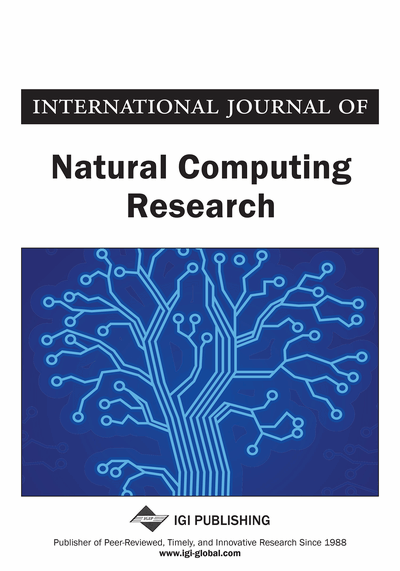# Innovative Genetic Algorithmic Approach to Select Potential Patches Enclosing Real and Complex Zeros of Nonlinear Equation

DOI: 10.4018/IJNCR.2017070102

## Abstract

In this article, an innovative Genetic Algorithm is proposed to find potential patches enclosing roots of real valued function f:R→R. As roots of f can be real as well as complex, the function is reframed on to complex plane by writing it as f(z). Thus, the problem now is transformed to finding potential patches (rectangles in C) enclosing z such that f(z)=0, which is resolved into two components as real and imaginary parts. The proposed GA generates two random populations of real numbers for the real and imaginary parts in the given regions of interest and no other initial guesses are needed. This is the prominent advantage of the method in contrast to various other methods. Additionally, the proposed ‘Refinement technique' aids in the exhaustive coverage of potential patches enclosing roots and reinforces the selected potential rectangles to be narrow, resulting in significant search space reduction. The method works efficiently even when the roots are closely packed. A set of benchmark functions are presented and the results show the effectiveness and robustness of the new method.
Article Preview
Top

## 1. Introduction

Most of the engineering problems are inherently nonlinear in nature leading to the problem of obtaining all roots of single nonlinear equations. Some such engineering problems are those concerned with stability of systems, vibrations, periodic processes, electrical circuits with alternating current sources, wave phenomena and so on. Solving such nonlinear equations involving transcendental terms is an ever-challenging problem to those seeking to obtain closely packed complex roots. The advent of high-speed digital computers gave us the opportunity to expand the search to find all roots (real and complex) of single nonlinear equations, from which one can select more useful roots. Furthermore, even a system of nonlinear equations can be reduced to a single equation, for which all roots can then be found.

The conventional numerical iterative methods to solve a nonlinear equation can be mainly categorized as open methods and interval based methods. Most of the open methods such as Newton-Raphson method are point based approaches with one or more initial guess points and they generally converge fast as described (Press, 2007; Goyal, 2007). But, the main drawback here is about having a proper initial guess for roots as well as number of roots (Burden & Faires, 2001; Traub, 1964). Interval based methods such as Bisection method, Regula-falsi method are robust but they converge slowly (Ortega et al., 2014). Both the above methods deliver only one root at one execution of the algorithm. Furthermore, these methods do not guarantee to find exhaustive roots in the region of interest (ROI). Additionally, to find a complex root, it is generally required that the initial guess must be complex (Antia, 2012; Krantz, 1999).

The Newton and Halley Method for Complex Roots was presented by Yau and Ben-Israel (1998). Pan and Zheng (2011) proposed a matrix method to find real and complex roots of polynomials by making use of approximating the roots as the eigen values of the companion or generalized companion matrix associated with an input polynomial. Oftadeh developed a cubically convergent iterative method for finding complex roots of nonlinear equations (Oftadeh et al., 2010). Gritton, proposed global homotopy continuation procedures for seeking all roots of a nonlinear equation by considering several nonlinear equations related to chemical engineering problems (Gritton, Seader et al., 2001). Method suggested by Pourrajabian et al. (2013) made use of Genetic algorithms for solving nonlinear algebraic equations. Ariyaratne et al. (2016) proposed a modified firefly algorithm to solve univariate nonlinear equations possessing complex roots. Ujevic (2007) suggested an iterative method for solving nonlinear equations. But, it is observed that most of these methods cannot effectively find the roots (especially complex roots), if they are closely packed.

## Complete Article List

Search this Journal:
Reset
Open Access Articles: Forthcoming
Volume 9: 4 Issues (2020): 1 Released, 3 Forthcoming
Volume 8: 4 Issues (2019)
Volume 7: 4 Issues (2018)
Volume 6: 2 Issues (2017)
Volume 5: 4 Issues (2015)
Volume 4: 4 Issues (2014)
Volume 3: 4 Issues (2012)
Volume 2: 4 Issues (2011)
Volume 1: 4 Issues (2010)
View Complete Journal Contents Listing